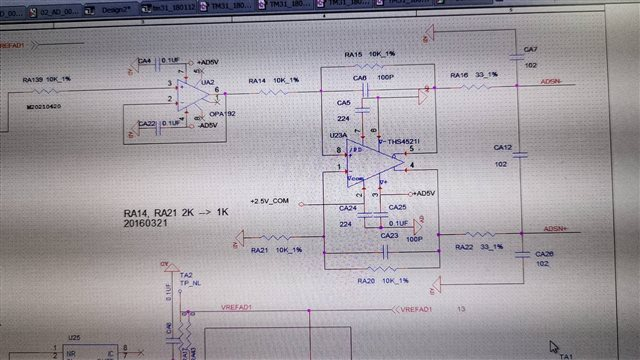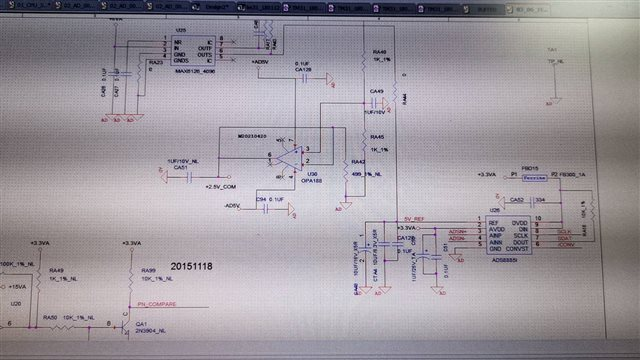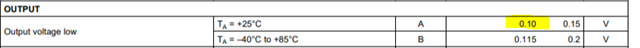If you have a related question, please click the "Ask a related question" button in the top right corner. The newly created question will be automatically linked to this question.

# ADS8885: there would be around +/-1000 value variation at the digital output when VINN/VINP input very close to Vref

Other Parts Discussed in Thread: THS4521

Hi,

We have a problem when using ADS8885 with THS4521 as ADC buffer.

When VINN/VINP input near to 4V and Vref=4.096V, there would be around +/-1000 value variation at the digital output.

This problem would be solved when the input be smaller like 3V or less. (only +/-7 value variation at the digital output.)

The problem also be found when input=5V and Vref=5V.

The power for THS4521 is always 5V.

My questions are what may cause this problem and how could we solve it?

Thank you.Best Regards,

Cindy

• Hi Cindy,

The common mode voltage is 1/2*Vref.  When Vref=4.096V, the common mode voltage will be 2.048V.  For Vin=4V, ADSN+=4.048V and ADSN- should be 0.048V.  However, the output of the THS4521 cannot go all the way to ground, 0V, due to the output voltage limitation.  The typical minimum output voltage is 100mV with 1kohm feedback resistors.  In your case, the feedback resistors are 10kohm, and the output can go lower, but will still have several millivolts.Change resistor values RA40 and RA45 so that the common mode voltage, +2.5V_COM, is 2.5V when using 4.096V reference.

Using RA40=1.37kohm and RA45=2.15kohm will set Vocm=2.5V with Vref=4.096V.

Regards,
Keith Nicholas